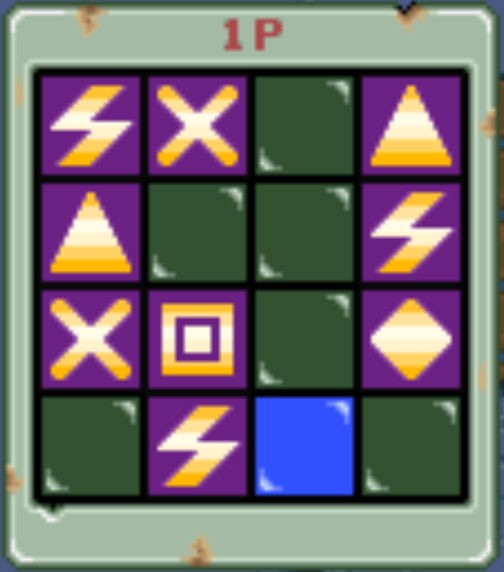Biker Mice from Mars Guides and Walkthroughs

This page here will share minor tidbits, tricks, cheats and hints about Biker Mice from Mars. As with many of the other guides on my website these are designed to be very google friendly. If you're stuck, and googling to get done a certain part of the game, that's hopefully how you found this guide!

Biker Mice from Mars Game Genie Codes (USA)

Biker Mice from Mars Pro Action Replay Codes (USA)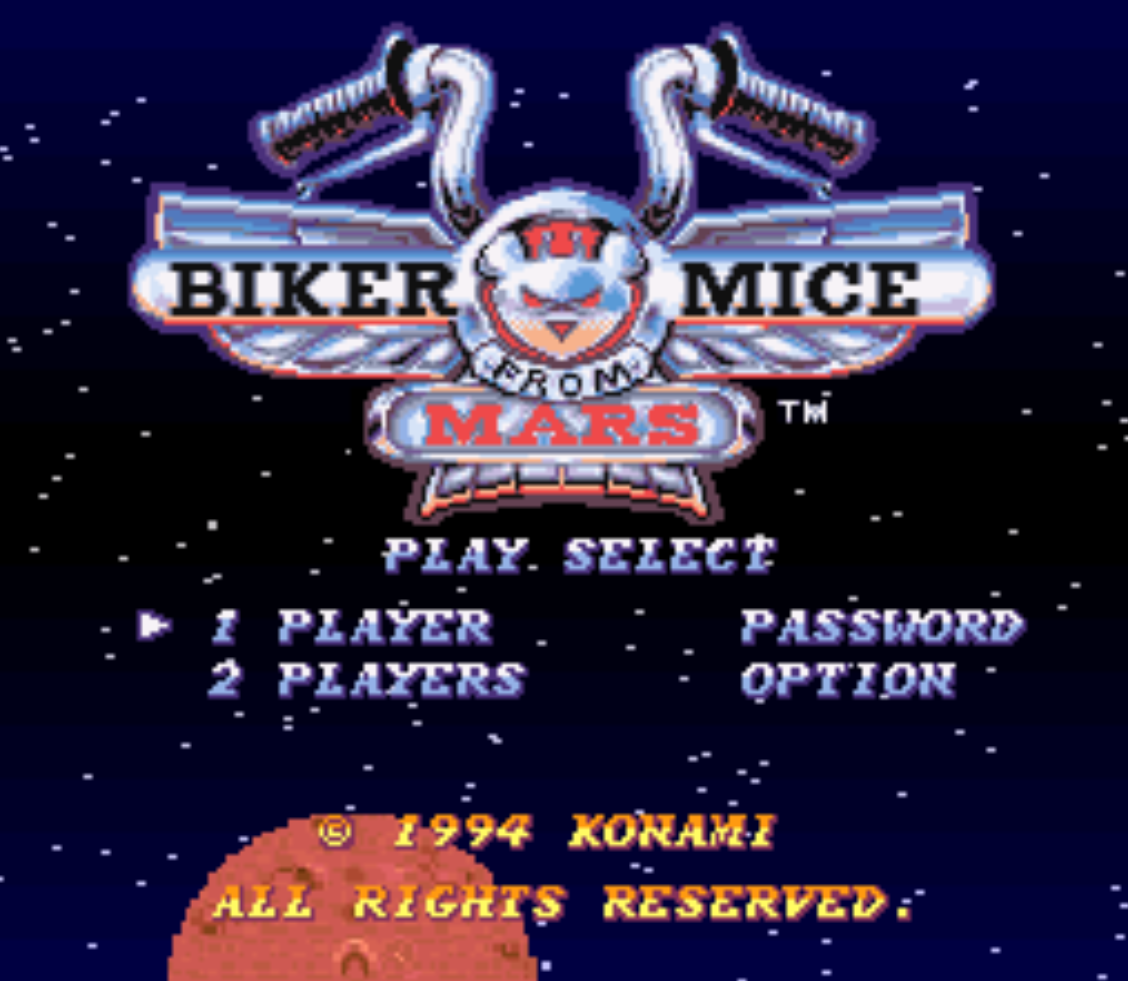Biker Mice From Mars Passwords

The following passwords can be entered into the game without Game Genie or Action Replay. Each password will perform a different task so keep an eye on which one you're using!

Start as Modo with all upgrades on Hard

Circle, Diamond, Diamond, Lightning
Circle, Blank, Square, Square
X, Square, Square, V
Blank, Lightning, Diamond, DiamondStart as Grease Pit with all upgrades

Diamond, X, Diamond, Triangle
Circle, Blank, Square, Diamond
Square, Square, Square, V
Blank, Triangle, Square, Circle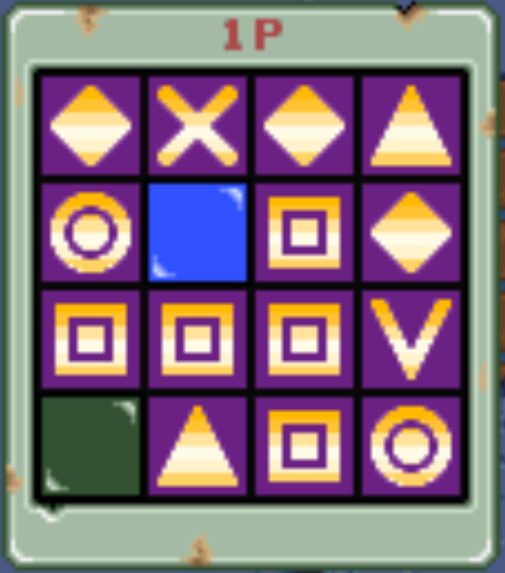Start as Karbunkle with all upgrades

Diamond, V, Circle, Triangle
Lightning Bolt, Blank, Square, Square
Square, Square, Square, V
Triange, Lightning Bolt, X, Circle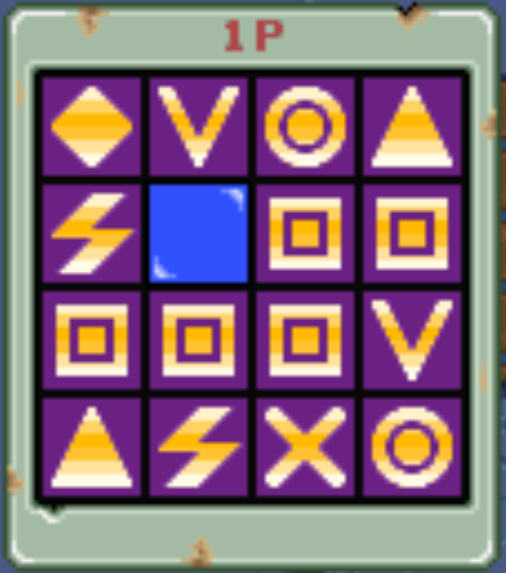Start as Throttle with all upgrades

Circle, Square, Circle, Lightning Bolt
X, Blank, Blank, Diamond
Square, Blank, Square, Diamond
Triangle, Triangle, Square, Diamond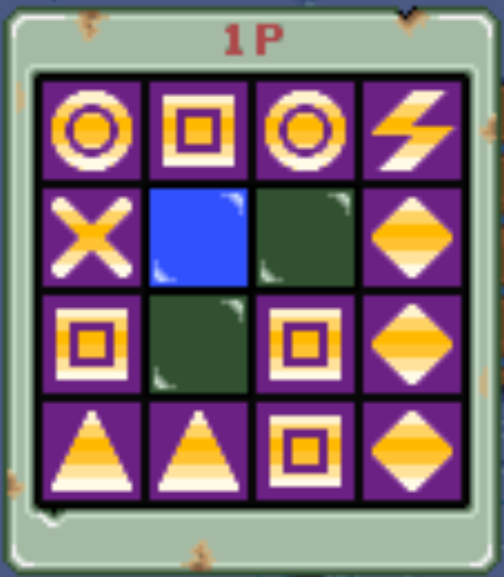Start as Vinnie with all upgrades

Circle, Diamond, Diamond, Triangle
V, Blank, Square, V
Square, Blank, Square, Diamond
Triangle, Triangle, Square, Diamond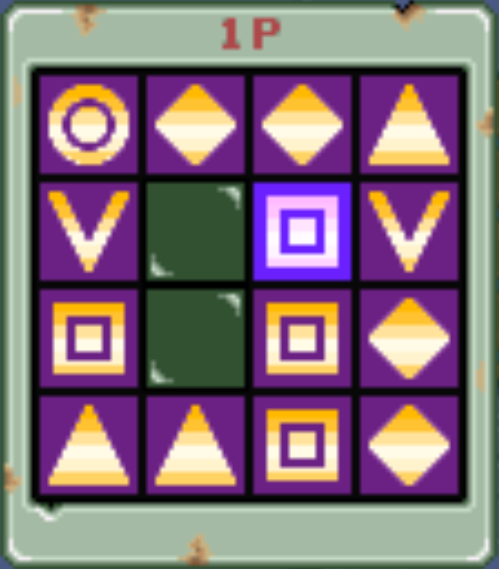Blank, Triangle, Square, Blank
X, Square, Blank, Circle
Blank, Square, Blank, X
Square, Diamond, Diamond, VUnlock Super Hard Difficulty

At the main menu on Controller 2 you'll want to input the following code to unlock the super hard difficulty. If you input the code successfully you'll hear a laugh that confirms correct entry.

Up, X, Left, Y, Down, B, Right, A, Up, X

These passwords are for Modo only and are seperated primarily by difficulty.

Modo Level (Easy 1)

Blank, X, Diamond, Lightning Bolt
Triangle, Blank, Blank, Triangle
Blank, Square, Blank, Circle
Lightning Bolt, Circle, Diamond, BlankModo Level (Easy 2)

Blank, Lightning Bolt, Blank, Circle
Lightning Bolt, Square, Blank, Diamond
X, Blank, Blank, Blank
Square, Lightning Bolt, Square, VModo Level (Normal 1)

Blank, Triangle, Circle, Triangle
Diamond, Blank, Blank, Triangle
X, Square, Blank, Triangle
Lightning Bolt, Lightning Bolt, X, Triangle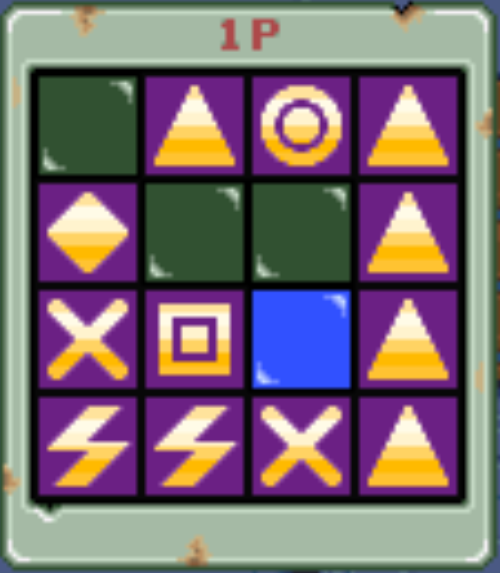Modo Level (Normal 2)

Blank, Lightning, Diamond, Blank
X, Square, Blank, Diamond
Square, Square, Blank, Diamond
Square, Circle, V, DiamondModo Level (Normal 3)

Blank, Diamond, Diamond, Lightning
Diamond, Square, Blank, Diamond
Triangle, Square, Square, Diamond
Circle, Blank, Blank, BlankModo Level (Normal 4)

Triangle, Triangle, Triangle, Circle
Square, Blank, Blank, V
Blank, Square, Square, Diamond
Blank, Circle, Circle, Circle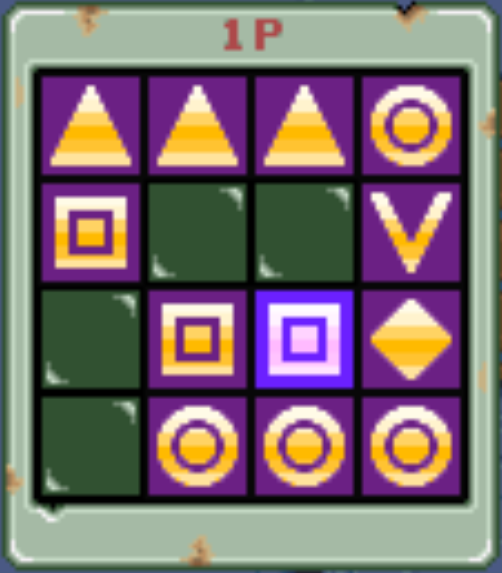Modo Level (Hard 1)

Circle, Triangle, Square, Lightning Bolt
Blank, Blank, Blank, Triangle
Triangle, Square, Blank, Diamond
Lightning Bolt, Lightning Bolt, Square, BlankModo Level (Hard 2)

Circle, Lightning Bolt, Circle, Blank
Lightning Bolt, Square, Blank, Diamond
X, Blank, Blank, X
Square,Circle, Square, VModo Level (Hard 3)

Circle, Diamond, V, Lightning Bolt
Circle, Square, Blank, Triangle
Square, Blank, Square, V
Circle, Lightning Bolt, Triangle, DiamondModo Level (Hard 4)

Lightning Bolt, X, Blank, Triangle
Triangle, Blank, Blank, Lightning Bolt
X, Square, Blank, Diamond
Blank, Lightning Bolt, Blank, Blank